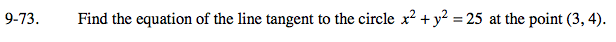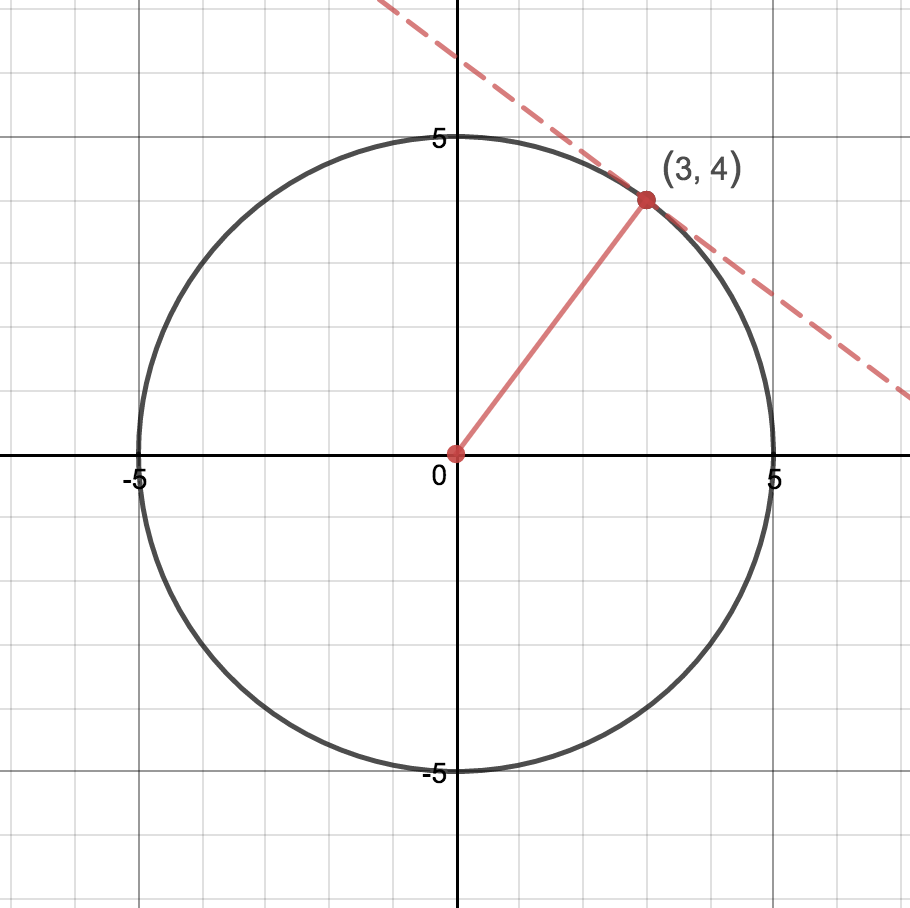### Home > AC > Chapter 16 > Lesson 16.9.2.1 > Problem9-73

9-73.The radius is perpendicular to the tangent line. Find the slope of the radius to help find the slope of the tangent line.

$m_{\text{radius}}=\frac{4}{3}\text{ } m_{\text{tangent}}=-\frac{3}{4}$

Use the given point and mtangent to find the equation.